Home » Services and Admin » ICT Service » Software » Introduction to Stata

# Introduction to Stata

## Introduction

Stata is among the most popular software packages for performing econometric analyses. It features a large set of econometric techniques and efficient data handling procedures. Users without any background in command-oriented software may find the first steps difficult, but once the basic principles are understood, the software allows for easy access to a wide set of different estimators.

Stata can be used in three different ways. In the interactive mode you directly type the commands in the command window. If you're unfamiliar with the syntax of a particular command you can execute it via menus as in any other Windows software. Newcomers may find this option particularly attractive. As you become more familiar with Stata you may want to work with do-files. They allow for fast execution of command sequences such that you work more efficiently. If you use the menus Stata generates code that you then can copy into the do-file.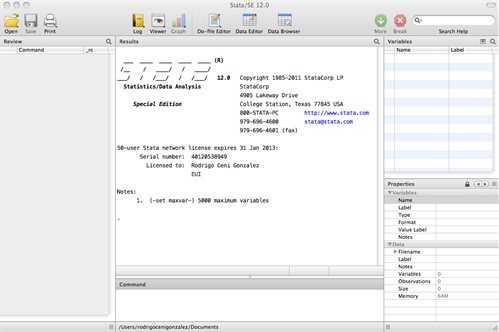Main types of variables: .dta data files; .do command files; .ado programs or commands; .hlp help files; and .gph graphs.

Load, open and save Stata format files (.dta):

First: set the memory (in the versions below 12)

set mem 200m

use "U:\Working\data\2011.dta", clear

(clear: delete all the data which the memory is using)

Third: work with the data base and save it:

save "U:\Working\data\2011.dta", replace

If you use the same directory you can fix it:

cd "U:\Working\data\"

and then

use "2011.dta", clear

and

save "2011.dta", replace

(saveold "2011.dta", replace)

in the version12 in order to use the database in older versions.

If you want to use some variables (month, gender, labor, wages) you can:

use year gender labor using "U:\Working\data\2011.dta"

## Data Description

To see the names of variables, type of variable (numeric or string):

describe (des)

To see the basic statistics (number of observations, mean, standard deviation, min, and max ) of each variable:

summarize (sum)

If you want the percentiles, the skewness and the kurtosis:

summarize, detail (sum, detail)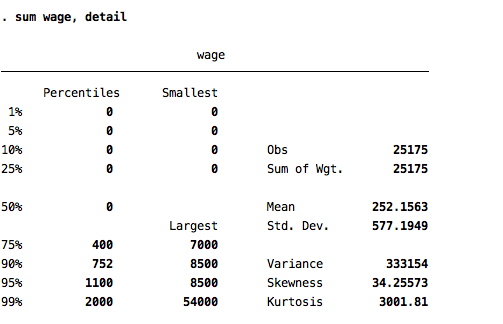If you want apply these commands for a set the variables you write the name of the variable after the command

sum labor, detail

If you want to see the frequency you can tabulate the variables (one way):

tabulate (tab) labor

Two ways:

tabulate (tab) gender labor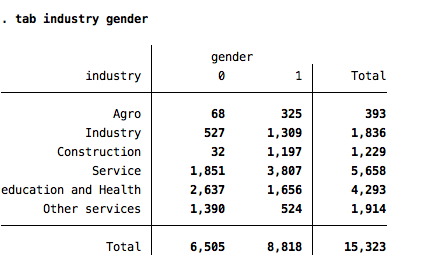Considering the missing observations

tab gender labor, missing (m)

Restricting for some group (e.g. only in June)

tab gender labor if month==6,m

## Basic Commands

If you want generate a new variable

Generate the logarithm of the wages

generate (gen) lwage=log(wage)

Generate a dummy variable (0 or 1) if the individual is unemploy (e.g in the labor variable it is the number 2)

gen unemploy=0

replace unemploy=1 if labor==2

or also to generate a dummy variable for each labor condition (you can generate with the name labor1, labor2, labor3…)

tab labor, g(labor)

another example generating a new variable:

gen quarter=.

replace quarter=1 if month>=1 | month<4

replace quarter=2 if month>=4 | month<7

replace quarter=3 if month>=6 | month<10

replace quarter=4 if month>=9 | month<=12

Generating a variable indicating some order for example by income:

sort income

gen id=_n

Other examples

gen abs_diff=abs(wage-taxes)

gen sqage=age^2

gen ones=1

Putting a new name:

rename sqage age2

Recoding:

recode gender 2=0

Using recode to create a new variable:

recode month (1/3=1) (4/6=2)(7/9=3)(10/12=4), gen(quarter)

Keeping and dropping cases:

drop if wage==0

keep if age>17

egen creates newvar of the optionally specified storage type equal to function(arguments). Here function() is a function specifically written for egen, as documented below or as written by users. Only egen functions may be used with egen, and conversely, only egen may be used to run egen functions.

egen sumwage=sum(wage)

egen meanwage=mean (wage), by (gender)

Other functions:

min Minimum value

max Maximum value

mean mean

median median

pctile percentile

sd standard deviation

Basic loop, generate a variable wage for each quarter:

foreach quarter in 1-4 {

gen wage`num'= wage if quarter==`num'

}

Another way:

forval x = 1/4 {

gen wage`x’=wage if quarter==`x’

}

Simpler linear regression:

reg lwage education age age2 gender

reg lwage education age age2 gender, robust (control by heterocedasticity)

reg lwage education age age2 gender, noconstant (without constant)

reg lwage education age age2 gender [aw=weight] (weighting)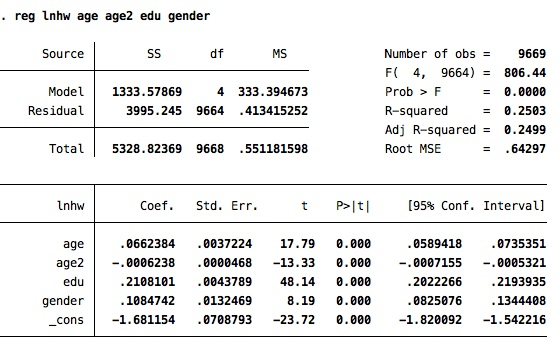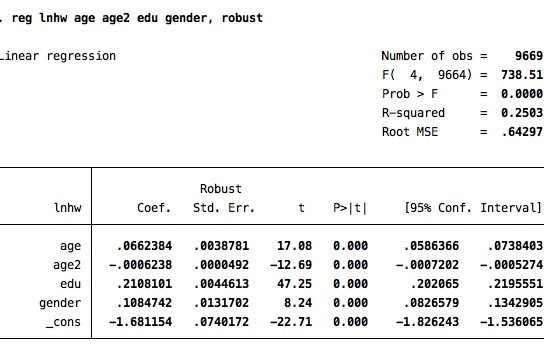postestimation:

predict lwage_est

( xb linear prediction; the default)

predict  res, r

There are more options in help regress_postestimation

Logistic regression:

logit success education age age2 gender

logit success education age age2 gender, robust (control by heterocedasticity)

logit success education age age2 gender, noconstant (without constant)

logit success education age age2 gender [aw=weight] (weighting)

logit success education age age2 gender, or (odds ratios)

postestimation:

predict yhat, pr

(probability of positive outcome, the default)

predict yhat, xb

( xb  linear prediction)

predict  res, r

(residuals)

There are more options in help logit_postestimation

Export regressions to Latex:

eststo: reg lwage education age age2 gender

eststo: reg lwage education age age2 if gender==1

eststo: reg lwage education age age2 if gender==0

estout, cells(b(star fmt(a3)) se(fmt(2) par)) starlevels(* 0.10 ** 0.05 *** 0.01)stats(r2 N, fmt(3 0)) style(tex) varlabels(_cons _cons)

## Graphics

Stata has a powerful and extensive graphics' package. Here I will present some examples but there are many examples and personalized tool that you can see with the command help:

### Dipersion graph

twoway (scatter lwage education)

(scatter lwage education)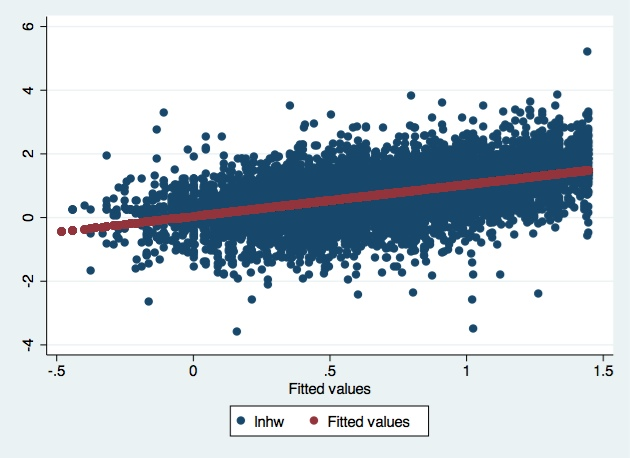### Dispersion graph by gender group

twoway (scatter lwage education), by(gender)

(scatter lwage education), by(gender)

### Dispersion graph with linear approximation

twoway (scatter lwage education) ///

(lfit lwage education) , ///

ytitle(Wages (Ln)) xtilte(Education)

### Histograms

hist education, freq

### Bar-Charts

graph bar education, by(gender)

graph bar gender, by(education)

Page last updated on 17 August 2017